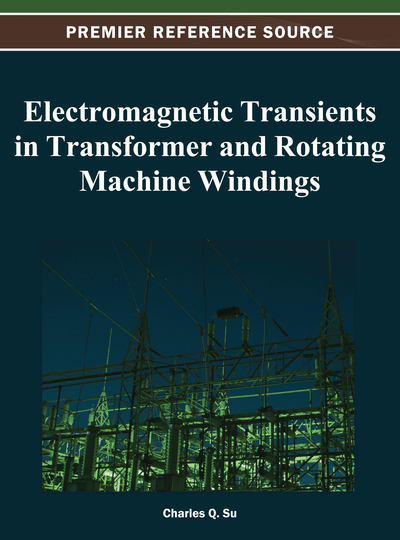# Transformer Insulation Design Based on the Analysis of Impulse Voltage Distribution

Jos A.M. Veens
DOI: 10.4018/978-1-4666-1921-0.ch011
OnDemand:
(Individual Chapters)
Available
\$29.50
No Current Special Offers

## Abstract

In this chapter, the calculation of transient voltages over and between winding parts of a large power transformer, and the influence on the design of the insulation is treated. The insulation is grouped into two types; minor insulation, which means the insulation within the windings, and major insulation, which means the insulation build-up between the windings and from the windings to grounded surfaces. For illustration purposes, the core form transformer type with circular windings around a quasi-circular core is assumed. The insulation system is assumed to be comprised of mineral insulating oil, oil-impregnated paper and pressboard. Other insulation media have different transient voltage withstand capabilities. The results of impulse voltage distribution calculations along and between the winding parts have to be checked against the withstand capabilities of the physical structure of the windings in a winding phase assembly. Attention is paid to major transformer components outside the winding set, like active part leads and cleats and various types of tap changers.
Chapter Preview
Top

## Estimation Of Impulse Voltage Distribution Via Winding Ratio And Oscillating Factor Method

The winding system of a power transformer consists generally of a minimum of two windings of different nominal voltage levels. The simplest example is a two-winding transformer with a fixed ratio, with (per phase) only one winding (in one part) for the LV winding and one winding (in one part) for the HV winding. Most of the time however, one of the two windings (usually the HV winding), has more than one part, because it needs to be adjustable in voltage. This means that a winding will have a discontinuity in electrical properties in the connection point between the two parts.

The impulse voltage distribution along a winding is usually not divided linearly according to the turns ratio, which is in contrast to the voltages at nominal frequency. The initial distribution is determined more by the series capacitances of the winding parts. The voltages tend to oscillate with a level that is approximately proportional to the difference between the initial capacitive voltage distribution and the final inductive voltage distribution, as shown in Figure 1.

For estimation purposes, and for a quick check of the correct behaviour of a transient model, a simple rule of thumb for the amplitude (peak-peak) of the oscillating voltage is assuming a multiplication factor of two, two times the nominal voltage.

The first winding type where this rule is applied is a layer winding. We take an example where the layer winding consists of six layers (of equal turns). See figure 2.

Nominal or induced voltages between the layer ends are: (100% / 6) x 2 layers = 33%.

But for impulse voltage distribution, we multiply this figure by a factor of two: 2 x 33% = 66%.

Another example configuration has one high voltage main winding and one regulating winding. It is assumed that the regulating winding has considerably higher series capacitance compared to the main winding. The turns of the regulating winding can be connected to be additive (plus tap position), or connected to be subtractive (minus tap position). In Figure 3, the four main positions, usually relevant for acceptance testing are given.

Transient voltages are also referred to as BIL, Basic Impulse Insulation Level, see IEEE Std, C57.12.90(2006). Transient voltage estimates or BIL estimates across the tap winding:

In tap Plus (a), the BIL level across the tap winding estimation: 2*60/(500+60)*100 = 21%.

## Complete Chapter List

Search this Book:
Reset